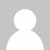Today’s Advent of Code challenge required us to count how many pairs of three coordinates represented possible triangles. The calculation itself is relatively straightforward – we just sort the sides and then see if the shortest two add up to greater than the longest.

I used regex again to pick the numbers out of the input file. One gotcha of the F# compiler’s type inference is that my code compiled and ran fine with the triangle sides as strings instead of integers since `isPossibleTriangle` works just fine with strings.

``````open System.Text.RegularExpressions
let isPossibleTriangle sides =
let sorted = sides |> Array.sort
sorted. + sorted. > sorted.

let input = System.IO.File.ReadAllLines (__SOURCE_DIRECTORY__ + "\\input.txt")

let getSides line =
Regex.Matches(line, "\d+") |> Seq.cast<Match> |> Seq.map (fun m -> int m.Value) |> Seq.toArray

input |> Seq.map getSides |> Seq.filter isPossibleTriangle |> Seq.length |> printfn "Part a: %d" // my answer = 982
``````

For the second part, it turns out that the sides of each triangle are not all on one line of the input file, but spread across three lines. This meant I wanted a `batch` function like MoreLINQ has, to batch the input into three lines. And I needed a `rotate` function that would take input like `[a;b][c;d]` and turn it into `[a;c][b;d]`. I also found myself wanting a simple helper to give numbers in the range 0 to n - 1.

I wouldn’t be surprised if F# already has some more elegant ways to solve these, so do let me know in the comments, but implementing them myself did at least give me the chance to use the array comprehension and slicing syntaxes:

``````let range (n:int) = { 0 .. (n - 1) }

let batch batchSize (array:'T[]) =
[for b in range (array.Length / batchSize) -> b * batchSize]
|> Seq.map (fun n -> array.[n..n+batchSize-1])

let rotate (a:'T[][]) =
[| for x in range a..Length -> [| for y in range a.Length -> a.[y].[x] |]  |]
``````

With these transformations in place, getting the sides is a case of batching, rotating, and then expanding out again with `Seq.collect`, which gets us back to a sequence of arrays of triangle side lengths which we can count in the same way as before:

``````let sides = input |> Array.map getSides |> batch 3 |> Seq.map rotate |> Seq.collect id
sides |> Seq.filter isPossibleTriangle |> Seq.length |> printfn "Part b: %d" // my answer = 1826
``````

Of course, once again this solution ends up a bit more verbose than the equivalent procedural code would have been, but if you take away the very generic helpers I had to make, the core solution is fairly simple. Full code available here.

As always, do check out the reddit thread for ingenious (and insane) solutions in other languages. Like last year, it does seem that Python is particularly well suited to these types of problems, with some of the most elegant and concise solutions for all three days so far being in Python.